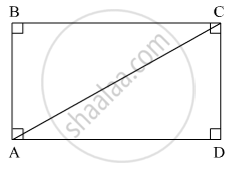SSC (English Medium) Class 8Maharashtra State Board
Share

# If Diagonal of a Rectangle is 26 Cm and One Side is 24 Cm, Find the Other Side. - SSC (English Medium) Class 8 - Mathematics

ConceptSome Special Parallelograms Concept of a Rectangle

#### Question

If diagonal of a rectangle is 26 cm and one side is 24 cm, find the other side.

#### Solution

Suppose ABCD is a rectangle.Here, segment AC is a diagonal and segment AD is one side of the rectangle ABCD.

l(AC) = 26 cm and l(AD) = 24 cm.

In right ∆ACD,

l(AC)2 = l(AD)+ l(CD)2               (Pythagoras theorem)

⇒ l(CD)= (26)2 − (24)2

⇒ l(CD)= 676 − 576 = 100

⇒ l(CD) =  sqrt(100) = 10 cm

Thus, the other side of the rectangle is 10 cm.

Is there an error in this question or solution?

#### APPEARS IN

Balbharati Solution for Balbharati Class 8 Mathematics (2019 to Current)
Chapter 8: Quadrilateral : Constructions and Types
Practice Set 8.2 | Q: 4 | Page no. 46
Solution If Diagonal of a Rectangle is 26 Cm and One Side is 24 Cm, Find the Other Side. Concept: Some Special Parallelograms - Concept of a Rectangle.
S